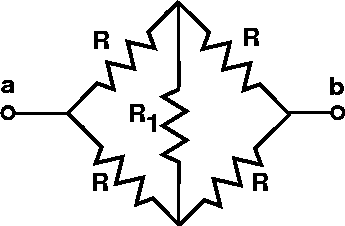# R1 350 Ohms: What Current Flows at 50V?

• x^2

## Homework Statement

What current flows through resistor R1 (350 Ohms) in the figure when the applied voltage is 50 V?V = IR ?

## The Attempt at a Solution

I would have thought the answer would be zero because I thought the potential at R1 is equal (but this was incorrect). What am I missing here? I guess I don't see how the potential at this point isn't equal, and I'm not sure how to solve it now knowing that it must not be the same.

x^2

Last edited:
I would have thought the answer would be zero because I thought the potential at R1 is equal (but this was incorrect). What am I missing here? I guess I don't see how the potential at this point isn't equal, and I'm not sure how to solve it now knowing that it must not be the same.

x^2

If the other R's are equal, then it is 0.

With 50V applied to AB, the terminals of each side of R1 would be 25V. No net voltage, no current.

If the other R's are equal, then it is 0.

With 50V applied to AB, the terminals of each side of R1 would be 25V. No net voltage, no current.

That's what I thought... I tried 0 A but it was incorrect. I'm going to talk to the prof. and see what is wrong.

Thanks!
x^2

That's what I thought... I tried 0 A but it was incorrect. I'm going to talk to the prof. and see what is wrong.

Thanks!
x^2

The correct answer is 0 A. There was a problem with the system which was why I was getting it wrong.

Thanks for the confirmation!
x^2